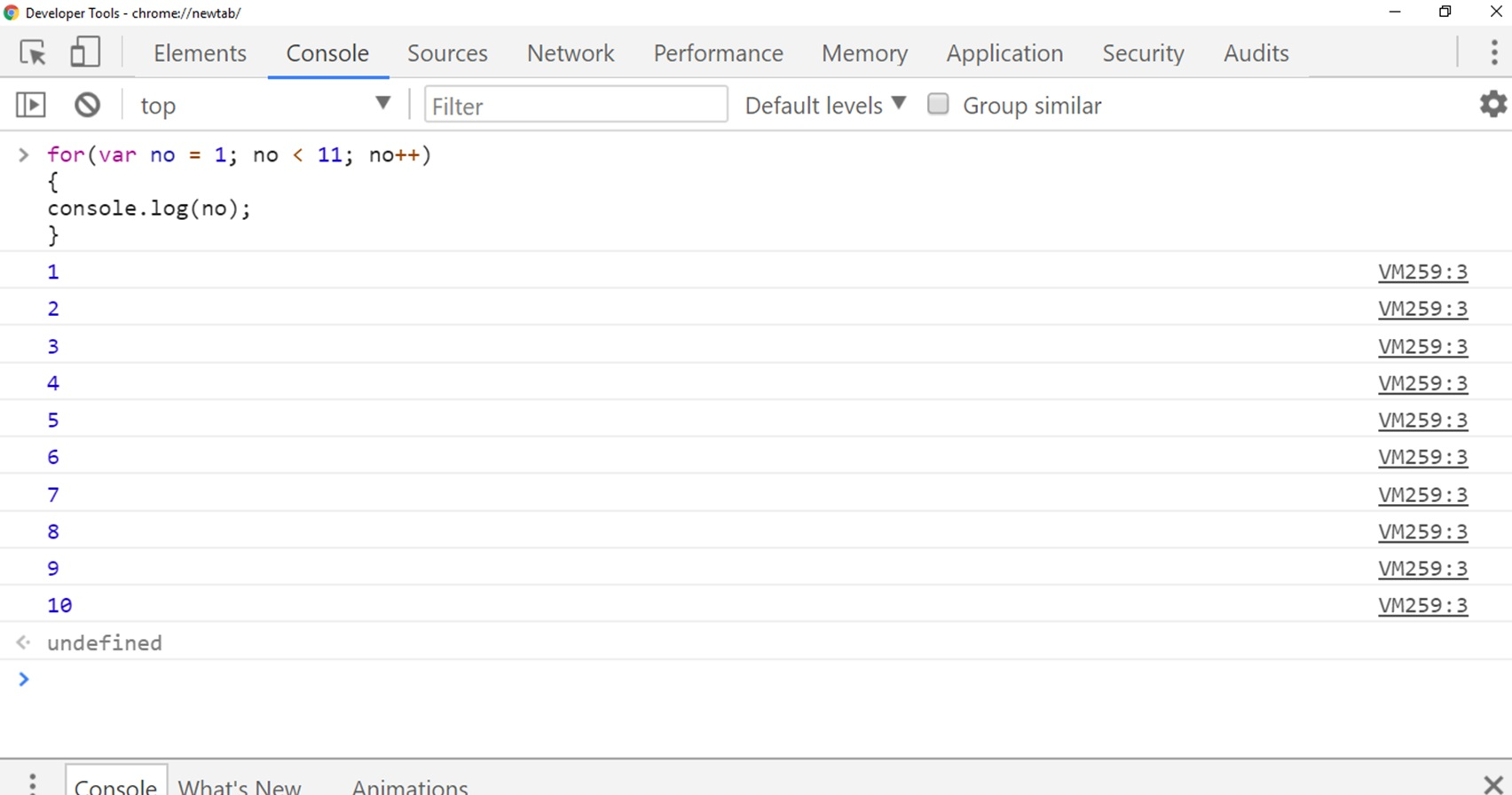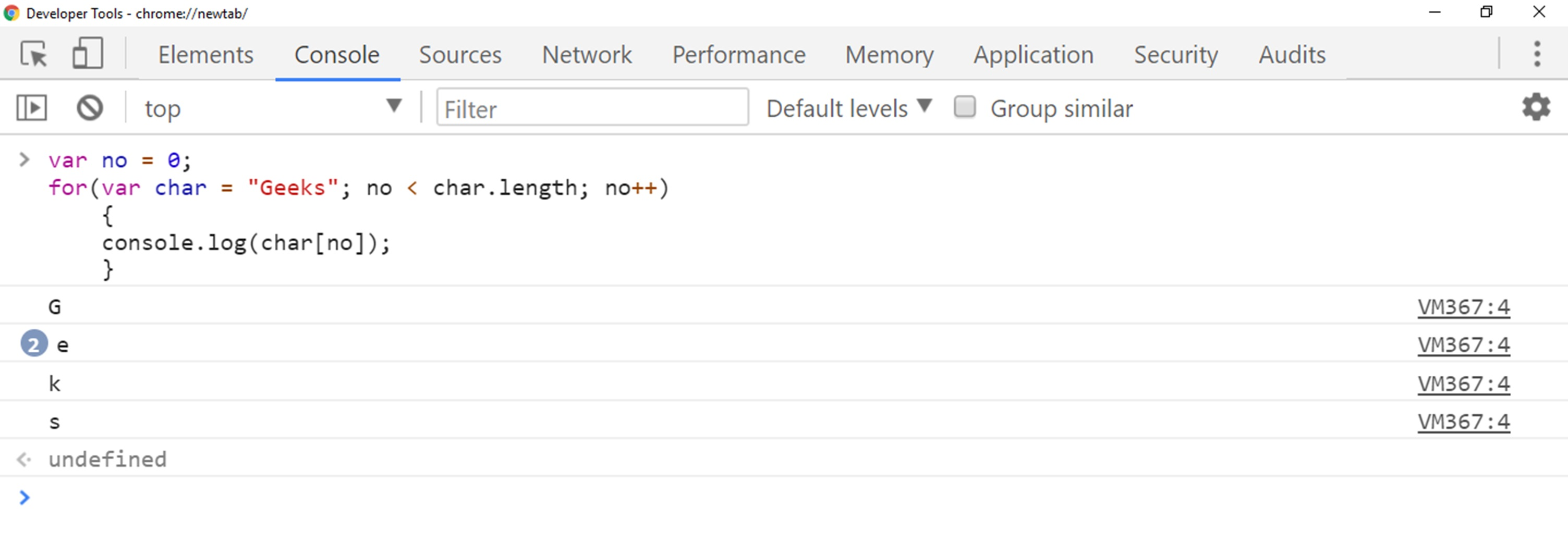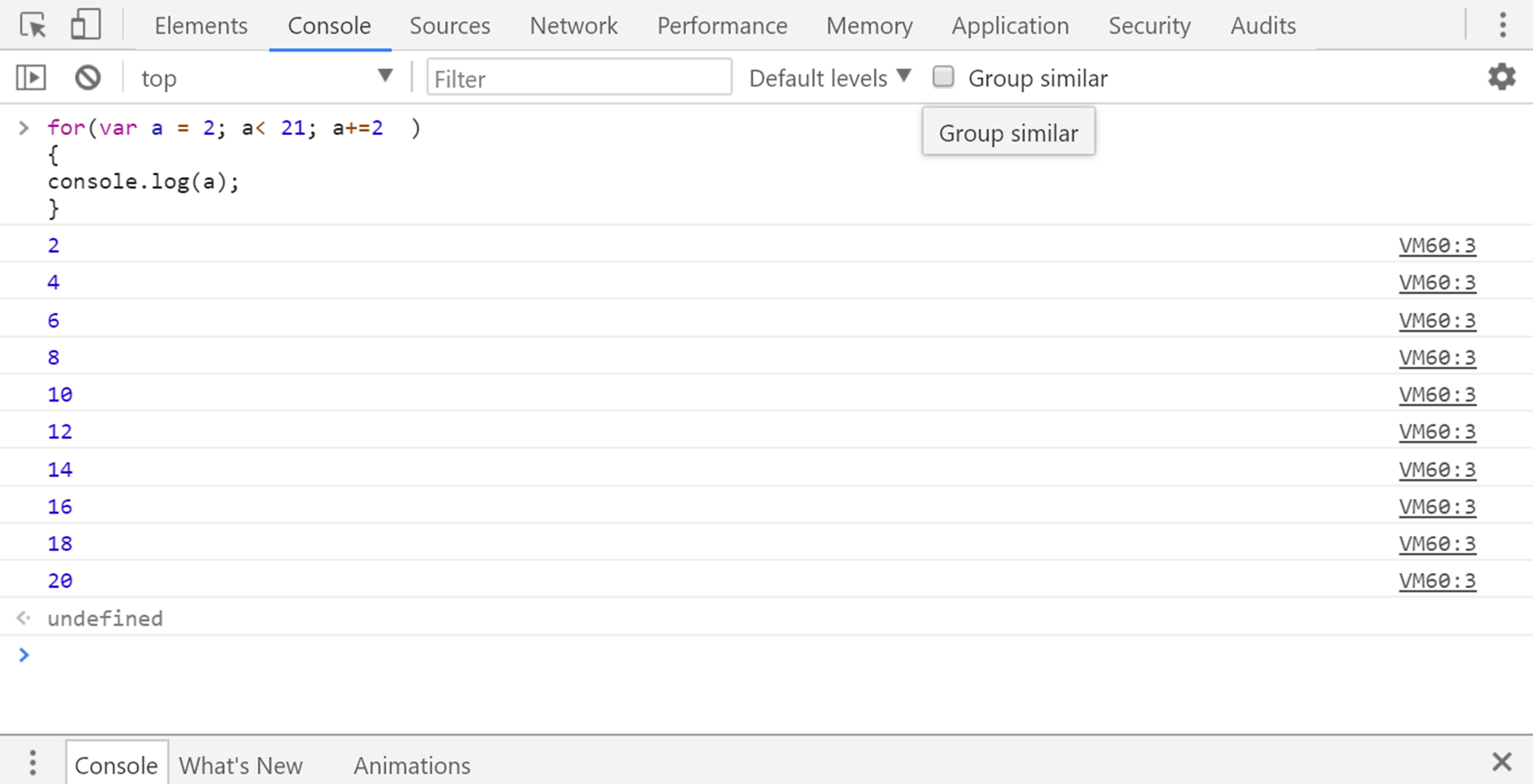# Lesson 12 For Loop in JavaScript

We are going one step deeper into JavaScript and going to learn another important topic called For Loop.
So in this lesson, we are going to learn for loop and its purpose.
At the end of this lesson, you will know how valid for loop can be written.
We will also compare and contrast while loop and for loop.
The objectives of this lesson are:

### Objectives

• Discuss the purpose of For Loop.
• Learn to write valid for loop.
• Differentiating while and for loop.

The ‘for’ loop is similar to ‘while’ loop and they both help us to reduce repetition of code. For and while loop both having different syntax.

## For Loop Syntax

The For Loop can be written as, ‘for’ keyword followed by parentheses and within parentheses, there will be three conditions. Here is the syntax below.

``````for (Loop Initializing Statement; Condition statement ; increment expression) {
//This block of code will be executed
}
``````

Before going into detail let’s try some simple example here.
So we are declaring a for loop which prints number from 1 to 10.

### JavaScript Code

``````for(var no = 1; no < 11; no++)
{
console.log(no);
}
``````

So when we run this code in JavaScript Console, then it will print number from 1 to 10.
Here is the output below.So you can notice in above code and output, we have written for keyword first to create for loop and after loop, we define three statement which is enclosed in parentheses.
So we are going to discuss these three statements in detail below.

### Loop Initialization:

Our first statement is the loop initialization, here we declare the starting counter of our code.
This statement will execute before the loop start.
For example, you can notice in above example where we initialize a variable with the name of ‘no’ keyword and set the starting value 1.

``````var no = 1;
``````

### Condition statement:

The condition statement is same that we saw in while loop.
So it defines and tests the condition whether it is true or false.
If this conditional statement is true then it will always execute curly braces statement.
You can notice in above example we have defined the statement, variable ‘no’ must less than 11.

``````no < 11;
``````

So the curly braces statement will execute every time if the condition statement is true.
In our scenario, our variable no is 1 and our condition statement is variable ‘no’ less than 11, so as long as ‘no’ variable is less than 11 it will execute curly braces statement.
So if the statement is no longer less than 11 then it will stop executing the code.

## Increment expression:

This one is one of the important statement for our loop, and this statement will execute at the end of each loop it will increase the variable ‘no’ value ever time code run, so it will update the counter variable every time code block run.

``````no++
``````

In our scenario, we are increasing the value of variable ‘no’ by 1 every time code run no++ is something like no=1+1, this statement not going to have a semicolon as we have in initializing and conditional statement.
So this is conceptually very much similar to the while loop, but the big difference is that our code is shorter and the other difference is that this variable count only exists inside the loop.
The variable for a While loop must be initialized outside of the loop.

### Example

So here is another example where we have declared a string “Geeks” and printing every character in a string but doing it with a for loop statement.

### JavaScript Code

Here is the JavaScript code for printing characters using for loopSo once again, you can see we don’t have to declare this char variable outside of a loop like we used to do in while loop, the reason is that it is pretty conventional the variable we declare inside loop do not exist for any other purpose but to live inside the loop.
Here is another example where we writing simple multiplication table 2 in JavaScript console.
So to write multiplication of 2 you need to first declare some variable and assign it 2 number, in our case we have declared variable ‘a’ and assign it value 2 and then in the condition statement we define a less than 21 it is because It will run code 20 times.
Here is the code of multiplication of 2 below.

## JavaScript Code

`````` for(var a = 2; a< 21; a+=2  )
{
console.log(a);
}
``````

Here is the output of multiplication of 2 JavaScript code.## Conclusion

In this lesson, we have learned to use for loop in JavaScript, and we have discussed in detail how we can construct loop, initialize loop statement and define the condition and incremental statement in loop